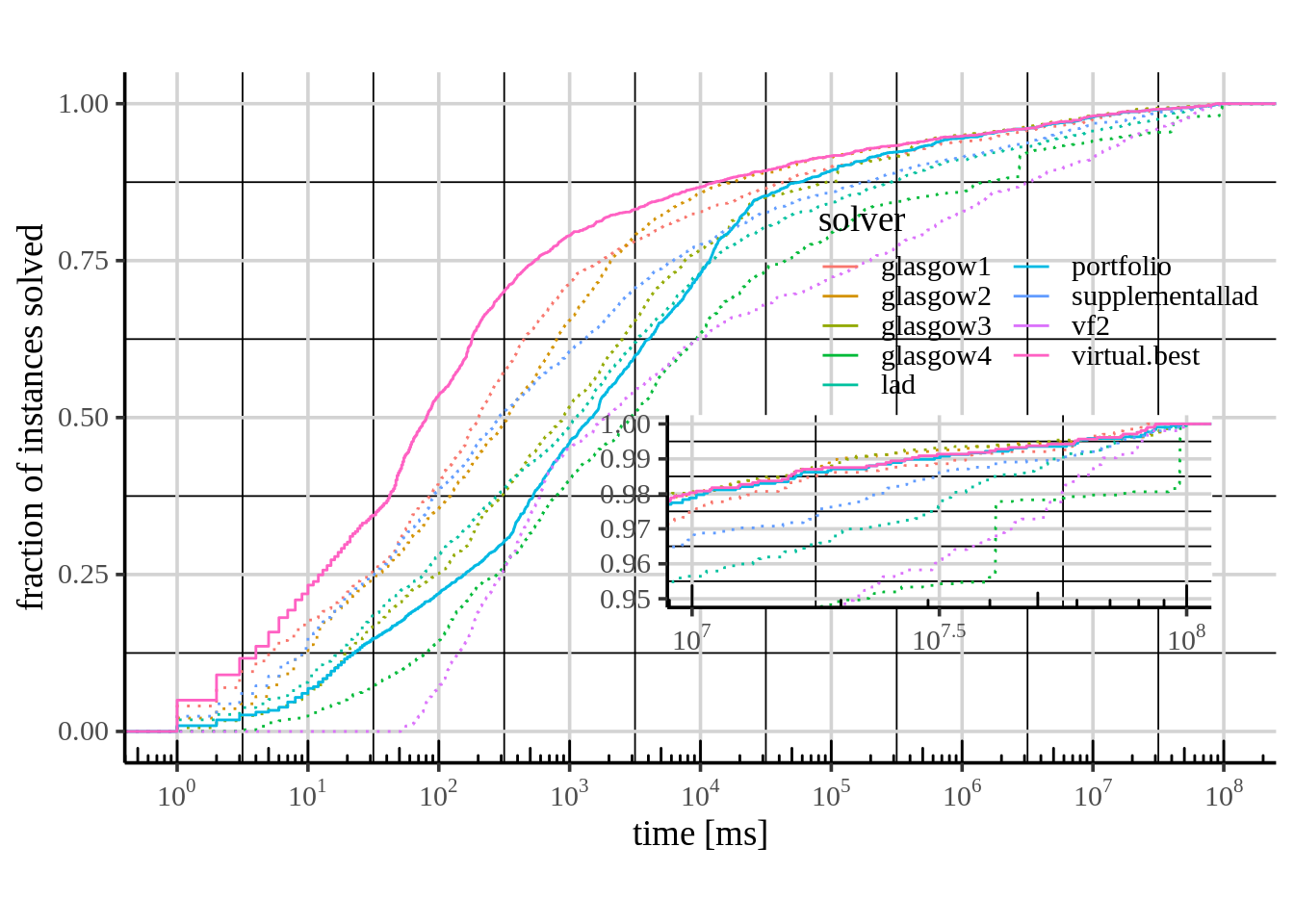## Description

Script to reproduce algorithm selection results from (Kotthoff, McCreesh, and Solnon 2016).

library(tidyr)
library(magrittr)
library(ggplot2)
library(ggthemes)
library(grid)
library(scales)
library(aslib)
library(llama)
library(randomForest)
library(parallelMap)
# Load dataset from file and convert to LLAMA object
dataset_raw <- parseASScenario("GRAPHS-2015")
dataset_structured <- convertToLlamaCVFolds(dataset_raw)

#Presolver 1: Filter instances solved by IncompleteLAD
dataset_notPresolved <- dataset_structured
presolved_ids <- dataset_raw$feature.runstatus$instance_id[dataset_raw$feature.runstatus$lad_features == "presolved"]
dataset_notPresolved$data <- subset(dataset_structured$data, !(dataset_structured$data$instance_id %in% presolved_ids))
dataset_notPresolved$best <- subset(dataset_structured$best, !(dataset_structured$data$instance_id %in% presolved_ids))

# Presolver 2: Filter instances solved by VF2 within 50 ms
dataset_hard <- dataset_notPresolved
dataset_hard$data <- subset(dataset_notPresolved$data, dataset_notPresolved$data$vf2 > 50)
dataset_hard$best <- subset(dataset_notPresolved$best, dataset_notPresolved$data$vf2 > 50)
dataset_hard <- cvFolds(dataset_hard)
cat("Count of all instances  =", nrow(dataset_structured$data), ", Count of hard instances =", nrow(dataset_hard$data)) # should be 5725 and 2336
## Count of all instances  = 5725 , Count of hard instances = 2336

## Train model

if (!file.exists("model_regr_hard.rds")) {
parallelStartSocket(4)
parallelLibrary("llama", "mlr")
start_time <- Sys.time()
system.time(model_regr_hard <- regressionPairs(makeLearner("regr.randomForest"), dataset_hard))
end_time <- Sys.time()
saveRDS(model_regr_hard, "model_regr_hard.rds")
cat("Training started at", format(start_time, "%X"), "and ended at", format(end_time, "%X"), "\n")
end_time - start_time
} else {
}
## Starting parallelization in mode=socket with cpus=4.
## Loading packages on slaves for mode socket: llama,mlr
## Mapping in parallel: mode = socket; cpus = 4; elements = 10.
## Training started at 08:23:52 and ended at 08:35:46
## Time difference of 11.91327 mins

## Algorithm selection results

resvbs = data.frame(model = "Virtual best solver",
mean.misclassification.penalty = mean(misclassificationPenalties(dataset_hard, vbs)),
solved = sum(successes(dataset_hard, vbs)),
mean.performance = mean(parscores(dataset_hard, vbs, factor = 1)),
median.performance = median(parscores(dataset_hard, vbs, factor = 1)))
ressb = data.frame(model = "Single best solver",
mean.misclassification.penalty = mean(misclassificationPenalties(dataset_hard, singleBest)),
solved = sum(successes(dataset_hard, singleBest)),
mean.performance = mean(parscores(dataset_hard, singleBest, factor = 1)),
median.performance = median(parscores(dataset_hard, singleBest, factor = 1)))
resrp = data.frame(model = "Pairwise random forest regression",
mean.misclassification.penalty = mean(misclassificationPenalties(dataset_hard, model_regr_hard)),
solved = sum(successes(dataset_hard, model_regr_hard)),
mean.performance = mean(parscores(dataset_hard, model_regr_hard, factor = 1)),
median.performance = median(parscores(dataset_hard, model_regr_hard, factor = 1)))
rbind(resvbs, resrp, ressb)

## ECDF plot

vbs.agg = aggregate(as.formula(paste("score~", paste(c("instance_id", "iteration"), sep="+", collapse="+"))), vbs(dataset_hard), function(ss) { ss })
vbs.agg$virtual.best = parscores(dataset_hard, vbs, factor = 1) vbs.agg$iteration = NULL
vbs.agg$score = NULL pmod = data.frame(instance_id = unique(model_regr_hard$predictions$instance_id), portfolio = parscores(dataset_hard, model_regr_hard, factor = 1)) perfs = subset(dataset_hard$data, TRUE, c("instance_id", dataset_hard$performance)) perfs = merge(perfs, vbs.agg, by = "instance_id") perfs = merge(perfs, pmod, by = "instance_id") wide = gather(perfs, "solver", "time", names(perfs)[-1]) wide$type = ifelse(wide$solver %in% c("virtual.best", "portfolio"), "pf", "alg") p.full = ggplot(wide, aes(x = time, col = solver, linetype = type)) + stat_ecdf() + scale_linetype_manual(values=c(3,1), guide = FALSE) + scale_x_log10(breaks = trans_breaks("log10", function(x) 10^x, n = 10), labels = trans_format("log10", math_format(10^.x)), limits = c(1, dataset_raw$desc$algorithm_cutoff_time-1)) + coord_cartesian(xlim = c(1, dataset_raw$desc$algorithm_cutoff_time-1), ylim = c(0,1)) + ylab("fraction of instances solved") + xlab("time [ms]") + annotation_logticks(sides = "b") + theme_tufte(base_family='Times', base_size = 14) + guides(col = guide_legend(ncol = 2, keyheight = .8)) + theme(legend.justification=c(1,0), legend.position=c(1,0.5), aspect.ratio = 0.6, axis.line = element_line(colour="black"), panel.grid = element_line(), panel.grid.major = element_line(colour="lightgray")) p.zoom = ggplot(wide, aes(x = time, col = solver, linetype = type)) + stat_ecdf() + scale_linetype_manual(values=c(3,1), guide = FALSE) + scale_x_log10(breaks = trans_breaks("log10", function(x) 10^x, n = 3), labels = trans_format("log10", math_format(10^.x)), limits = c(1, dataset_raw$desc$algorithm_cutoff_time-1)) + coord_cartesian(xlim = c(dataset_raw$desc$algorithm_cutoff_time/10, dataset_raw$desc\$algorithm_cutoff_time-1),
ylim = c(.95,1)) +
annotation_logticks(sides = "b") +
theme_tufte(base_family='Times', base_size = 14) +
theme(legend.position="none",
axis.title.x=element_blank(), axis.title.y=element_blank(),
panel.background = element_rect(fill='white', colour = "white"),
axis.line = element_line(colour="black"),
panel.grid = element_line(),
panel.grid.major = element_line(colour="lightgray"))
vp = viewport(width = 0.5, height = 0.3, x = 0.7, y = 0.42)
print(p.full)
print(p.zoom, vp = vp)## Source code

Kotthoff, Lars, Ciaran McCreesh, and Christine Solnon. 2016. “Portfolios of Subgraph Isomorphism Algorithms.” In LION 10.